# Online Civil Engineering Test - Previous UPSC Exam Papers Test 4Loading Test...

Instruction:

• This is a FREE online test. DO NOT pay money to anyone to attend this test.
• Total number of questions : 20.
• Time alloted : 30 minutes.
• Each question carry 1 mark, no negative marks.
• DO NOT refresh the page.
• All the best :-).

1.

The slenderness ratio of lacing bars should not exceed :

A.
 100B.
 200C.
 145D.
 1802.

A sample of ground water at a pH of 7.0 contains 122 mg/l of bicarbonates. What is the alkalinity of this water (in terms of CaC0 ) ?

A.
 120 mg/1B.
 60 mg/1C.
 100 mg/1D.
 200 mg/l3.

Soil at a site consists of two layers. The top layer has permeability k units and bottom layer has permeability 5k units. If the thickness of both the layers is equal, then what is the average permeability in the vertical direction ?

A.
 3k unitsB.
 (5/3)k unitsC.
 (6/5)k unitsD.
 (5/6)k units4.

What is the maximum bending moment at mid-span of the beam given below ?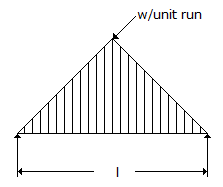A.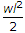B.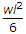C.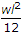D.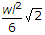5.

In the case of panel wall subjected to horizontal loads at right angles to the plane of the wall, with the mortar not leaner than M1 type, tensile stress in bending in the vertical direction may be allowed to the extent of

A.
 0.4 kg/cm2B.
 0.7 kg/cm2C.
 1.0 kg/cm2D.
 1.2 kg/cm26.

A particle of mass M is attached to a light horizontal wire which is stretched tightly between two fixed ends with a tension T. If a and b are the distances of the particle from the two ends, then the period of a small transverse oscillation of the particle is given by

A.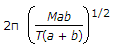B.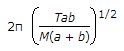C.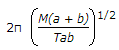D.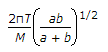7.

Which of the following measures are resorted to for strengthening masonry walls ?
1. Provide cross walls.
2. Pre-stressing.
3. Provide gunite slab on the surface(s) of walls.
4. Provide buttresses.
Select the correct answer using the code given below :

A.
 1, 3 and 4B.
 1 and 2C.
 2 and 3D.
 1, 2, 3 and 48.

The correct sequence of the increasing order of the disturbance to soil samples obtained from chunk, piston, split spoon and remolded sampler is

A.
 piston sampler, chunk sampler, split spoon sampler, remolded samplerB.
 chunk sampler, piston sampler, split spoon sampler, remolded samplerC.
 piston sampler, chunk sampler, remolded sampler, split spoon samplerD.
 chunk sampler, piston sampler, remolded sampler, split spoon sampler9.

Which of the following data would be required for the design of progressive type computerised traffic signal system in a series of intersection on a busy street ?
1. Volume of fast moving traffic
2. Volume of pedestrain traffic
3. Volume of slow traffic
4. Approach speed of fast moving traffic.
Select the correct answer using the codes given below :

A.
 1, 2, 4 and 5B.
 1, 2, 3 and 4C.
 2, 3, and 5D.
 1, 3, 4 and 510.

If the mean temperature of Sun's surface 6000 K and λm of its radiation is 0.5 what is the mean temperature of earth surface for which λm is 10.0 μm, according to Wien's Displacement Law in Remote Seniror Concept ?

A.
 25 °CB.
 28 °CC.
 27 °CD.
 30 °C11.

The changes that take place during the process of consolidation of a saturated clay would include

A.
 an increase in pore water pressure and an increase in effective pressureB.
 an increase in pore water pressure and a decrease in effective pressureC.
 a decrease in pore water pressure and a decrease in effective pressureD.
 a decrease in pore water pressure and an increase in effective pressure12.

Ratio of the width of the car parking area required at kerb for 30° parking relative to 60° parking is approximately

A.
 0.5B.
 0.7C.
 0.8D.
 213.

Under load, the void ratio of a submerged saturated clay decreases from 1.00 to 0.92. What will be the ultimate settlement of the 2 m thick clay due to consolidation ?

A.
 20 mmB.
 40 mmC.
 80 mmD.
 160 mm14.

A plane pin-jointed truss is shown in the figure. The force resisted by the member AD is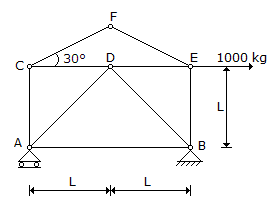A.
 zeroB.
 5002 kg. (compression)C.
 5002 kg. (tension)D.
 10002 kg. (tension)15.

If a sluice gate produces a change in the depth of water from 3.0 m to 0.6 m, then the force on the gate is about

A.
 9.5 kN/mB.
 19.0 kN/mC.
 38.0 kN/mD.
 76.0 kN/m16.

A saturated clay stratum of thickness 10 m, bounded on top and bottom by medium coarse sand layers, has a coefficient of consolidation of 0.002 cm2/s. If this stratum is subjected to loading, it is likely that it would undergo 50% of its primary consolidation in

A.
 1136 daysB.
 227 daysC.
 284 daysD.
 568 days17.

Due to horizontal pull of 60 kN at C, what is the force induced in the member AB?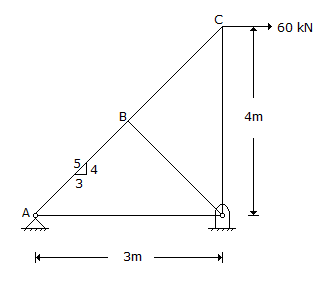A.
 0B.
 40 kNC.
 80 kND.
 100 kN18.

The Trap used for a water closet is called

A.
 gully trapB.
 p-trapC.
 intercepting trapD.
 anti-siphon trap19.

A section of a solid circular shaft with diameter D is subjected to bending moment 'M' and torque (T). The expression for maximum principal stress at the section is

A.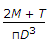B.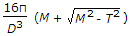C.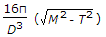D.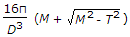20.

Consider the following steps :
1. Driving sheet piles surrounding a vibration-receiving structure.
2. Digging a trench around a source of vibration.
3. Placing rubber mountings between a machine causing vibration and its base. Active isolation of vibration can be achieved by

A.
 1 and 2B.
 1 and 3C.
 2 and 3D.
 3 alone